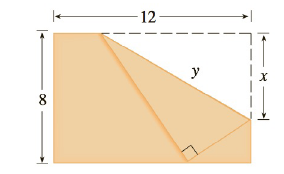Chapter 3.7, Problem 73E

Chapter
Section
Textbook Problem

# The upper right-hand corner of a piece of paper, 12 in. by 8 in., as in the figure, is folded over to the bottom edge. How would you fold it so as to minimize the length of the fold? In other words, how would you choose x to minimize y?To determine

To choose:

The value of x to minimize y

Explanation

1) Concept:

If f''x>0 if and only if fx is the absolute minimum value, where f'x=0

2) Calculation:

The upper right hand corner of a piece of paper, 12 in. by 8 in.

We would minimize the length of the fold that means

fx=y2

By using Pythagorean Theorem In DCB

y2=z2+x2

So, fx=z2+x2

By using Pythagorean Theorem

AC=x2-8-x2

AC=x2-64+16x-x2

AC=16x-4

AC=4x-4

Since CDE ~ BCA, we have

zx=84x-4

z=8x4x-4

### Still sussing out bartleby?

Check out a sample textbook solution.

See a sample solution

#### The Solution to Your Study Problems

Bartleby provides explanations to thousands of textbook problems written by our experts, many with advanced degrees!

Get Started

#### Find the remaining trigonometric ratios. 32. cosx=13,x32

Single Variable Calculus: Early Transcendentals, Volume I

#### What is the lowest score in the following distribution?

Essentials of Statistics for The Behavioral Sciences (MindTap Course List)

#### In Exercises 4562, find the values of x that satisfy the inequality (inequalities). 49. 6 x 2 4

Applied Calculus for the Managerial, Life, and Social Sciences: A Brief Approach

#### For what values of p does the series converge?

Study Guide for Stewart's Multivariable Calculus, 8th

#### Identify the threats to internal validity for pre-post designs.

Research Methods for the Behavioral Sciences (MindTap Course List)

#### Solve each equation. x436=35x2

College Algebra (MindTap Course List)